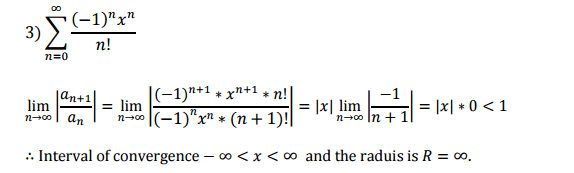# Power series

• I
I've 2 questions
1) Why do we take absolute of the power series?
2) I don't get why the interval of convergence is from -inifinity to +infinity. You can find the problem below.#### Attachments

PeroK
Homework Helper
Gold Member
2020 Award
For your first question, look up the "ratio test".

If the series converges for all ##x## then by definition the radius of convergence is ##\infty##.

FactChecker
Gold Member
I've 2 questions
1) Why do we take absolute of the power series?
That is called the ratio test of convergence. (https://en.wikipedia.org/wiki/Ratio_test) The absolute value is all you need to test convergence.
2) I don't get why the interval of convergence is from -inifinity to +infinity.
It converges if the absolute value of the limit is less than 1. In this case, the limit of the ratio is 0 regardless of the value of x. So it converges for any value of x.

That is called the ratio test of convergence. (https://en.wikipedia.org/wiki/Ratio_test) The absolute value

I've seen different problems with the ratio test and they didn't use absolute but when it comes to power series, they use it. Why?

PeroK
Homework Helper
Gold Member
2020 Award
I've seen different problems with the ratio test and they didn't use absolute but when it comes to power series, they use it. Why?

If you don't take the absolute value and the series has either alternating terms or at least infinitely many positive and negative terms, then the limit won't exist - unless it's 0. The test is better with the absolute value.

•FactChecker
Mark44
Mentor
I've seen different problems with the ratio test and they didn't use absolute but when it comes to power series, they use it. Why?
A power series is a series in powers of a variable such as x. The Ratio Test requires that all terms be positive, but with a variable, some terms could be negative, if x is negative. The problems you've seen that didn't use absolute values were almost certainly series in which all the terms were positive, such as ##\sum_{n = 1}^\infty \frac 1 {n^2 + 1}##.

•FactChecker